# ETAA Math Final

#### Numerical Sequences

For Questions #1-#4, find the missing number in each numeric sequence.

#### Plug In PEMDAS

For Questions #5-#6, substitute the numbers for the variables, simplify, and find the numeric solution.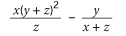For Questions #7-#8, substitute the numbers for the variables, simplify, and find the numeric solution.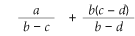For Questions #9-#10, substitute the numbers for the variables, simplify, and find the numeric solution.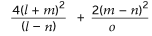For Questions #11-#12, substitute the numbers for the variables, simplify, and find the numeric solution.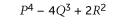For Questions #13-#14, substitute the numbers for the variables, simplify, and find the numeric solution.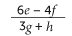For Questions #15-#16, substitute the numbers for the variables, simplify, and find the numeric solution.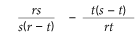#### Linear Equations

For Questions #17-#22, Match the Linear Equation expression in the question to the identical linear equation in the answers.

17.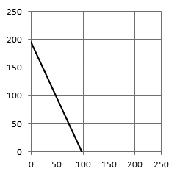A. one hundred and sixteen more than twice a number
B. y = 2x + 196
C.D. one hundred and ninety more than the opposite of a number
ABCD

18.A. Eighty nine more than a number
B. y = -3x + 89
C. Eighty nine less than three times a number
D.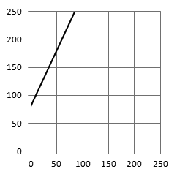ABCD

19. y = -4x + 113

A.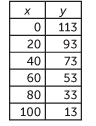B. One hundred thirteen more than a number
C.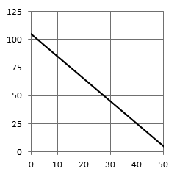D. One hundred thirteen more than four times the opposites of a number.
ABCD

20. One hundred and twenty four more than twice a number

A.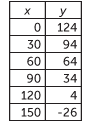B. y = x + 124
C.D. y = 2x + 124
ABCD

21.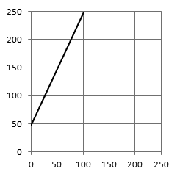A. y = -2x + 51
B.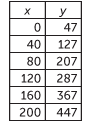C. y = x + 53
D. Forty seven more than a number.
ABCD

22. Eight nine more than four times the opposite of a number

A. y = -4x +89
B.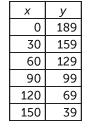C.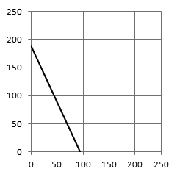D.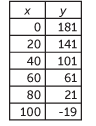ABCD

#### Dimensional Analysis

For Questions #23-#27, apply the relationships between the variables of the equation to determine the correct answer.

23.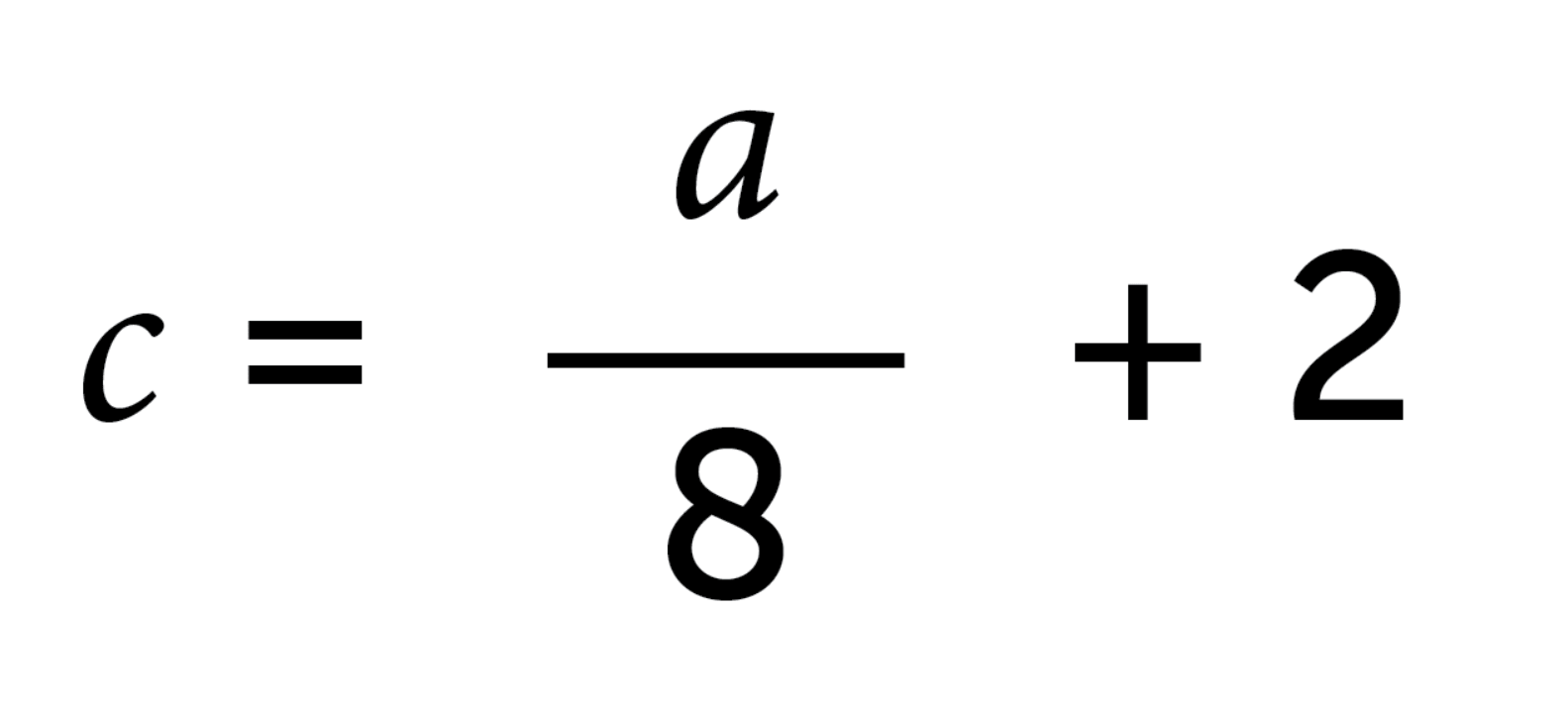Which of the following statements is true for the formula above?
A. When the value of a is greater than –8, c is positive.
B. When the value of a is less than –16, c is positive.
C. When the value of a is 0, c is negative.
D. When the value of a is greater than –16, c is negative.
ABCD

24.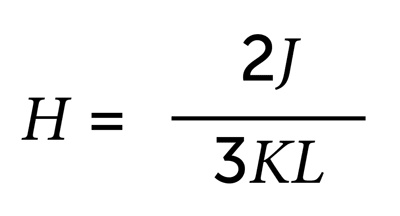In the formula above, if H remains constant, which of the following statements could be true?
A. J increases and K and L remain constant.
B. L increases and J and K remain constant.
C. J and L increase and K remains constant.
D. K and L decrease and J remains constant.
ABCD

25.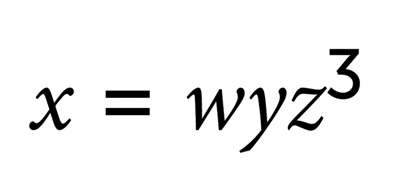In the formula above, if w is negative and y is positive, which of the following statements could be true?
A. If z is negative, then x is negative.
B. If z is negative, then x is positive.
C. x, y, and z are all negative.
D. If z is positive, then x is positive.
ABCD

26.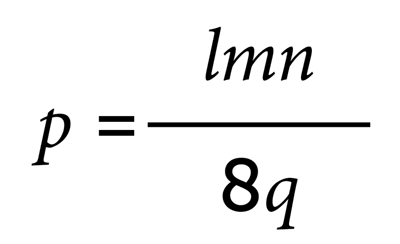In the formula above, if q is negative and m and n are different signs, which of the following statements is true?
A. If p is positive, then l is negative.
B. If p is negative, then l and q are opposite signs.
C. If l is positive, then p is positive.
D. If l is negative, then p and q are opposite signs.
ABCD

27.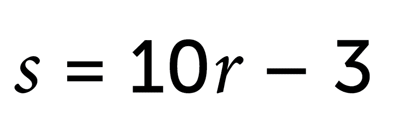Which of the following statements is true for the formula above?
A. If r = 0, then s = 0.
B. If r < 0, then s is positive.
C. If r > 3/10, then s is negative.
D. If r < 3/10, then s is negative.
ABCD

#### Variable Flip

28.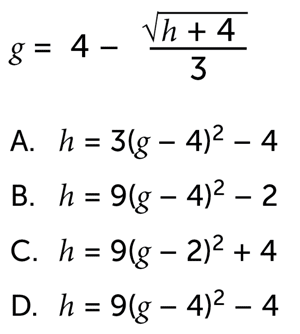ABCD

29.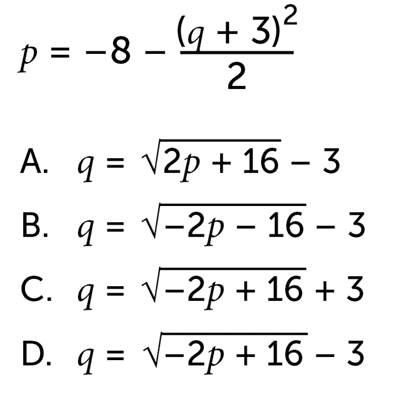ABCD

30.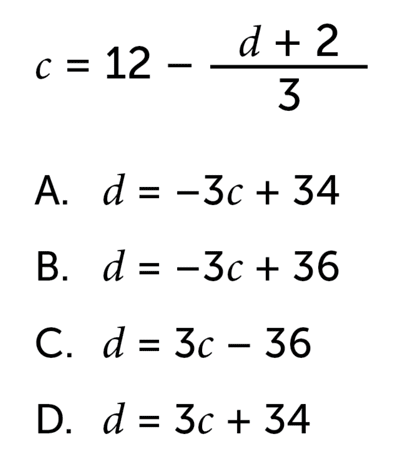ABCD

31.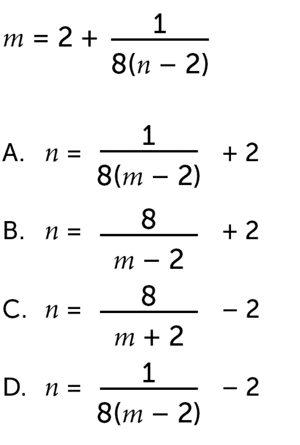ABCD

32.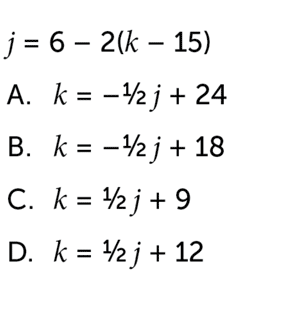ABCD

#### STOP

If you finish before time is called, you may check your work on this section only.
Do not turn to any other section.
Unauthorized copying or reuse of any part of this page is illegal.